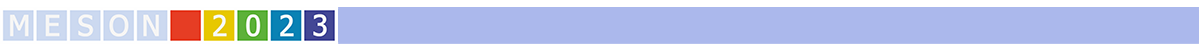#MESON2023

Jun 22 – 27, 2023
Auditorium Maximum
Europe/Warsaw timezone

## Light meson decays at BESIII

Jun 22, 2023, 3:45 PM
20m
Medium lecture hall (B) (Auditorium Maximum)

Parallel

### Speakers

The world's largest sample of $J/\psi$ events accumulated at the BESIII detector offers a unique opportunity to investigate $\eta$ and $\eta^\prime$ physics via two-body $J/\psi$ radiative or hadronic decays. In recent years the BESIII experiment has made significant progresses in $\eta/\eta^\prime$ decays. A selection of recent highlights in light meson spectroscopy at BESIII are reviewed in this report, including the observation of $\eta^\prime\rightarrow\pi^+\pi^-\mu^+\mu^-$, observation of the cusp effect in $\eta^\prime\rightarrow\pi^0\pi^0\eta$, search for CP-violation in $\eta^\prime\rightarrow\pi^+\pi^-e^+e^-$, as well as the precision Dalitz plot analysis of $\eta\rightarrow\pi^+\pi^-\pi^0$.